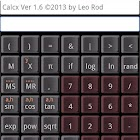All Android applications categories

All Android games categories# AndrCalcx

by: 15 6.8

6.8 Users
rating

## Screenshots

Description

Direct calculator with scrolling keyboard, 2D/3D graphics and anaglyph
2D graphics (Cartesian / polar coordinates), 3D graphics(spherical / parametric coordinates) and anaglyph.
example: 34*(57+22)-sin(M02/3)
Including: nth degree polynomial equation, numeric, integral and differential eq., polygons, statistics and financial.
Constants:pi, e and any integer or floating number.
Variables: memories M0 to M12, graphics (See Help)

Operators: + - * / ^ > < = & !
Functions: if(exp,exp1,exp2) Examples: if(3 > 4,1,2) if(M5 = 4,100,0)
sin() cos() tan() asin() acos() atan()
sinh() cosh() tanh() asinh() acosh() atanh() log() ln() rand()
abs() sqrt()

from 15 reviews

"Good"

6.8